Next: About this document Up: No Title Previous: No Title

# Introduction

There are many approaches to conventional Euclidian scattering theory. In this exposition an essentially microlocal view is adopted. Apart from its intrinsic interest this is intended as preparation for later generalization, to more complicated geometric settings. In fact, the treatment given here extends beyond the usual confines of scattering theory in that the spectral and scattering theory, at least the elementary part, is covered for the Laplacian associated to a `scattering metric' on any compact manifold with boundary.

By a scattering metric on a compact manifold with boundary, X, we shall mean a Riemann metric on the interior of X which can be brought to the form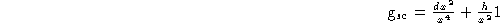whereis a boundary defining function and h is a smooth symmetric 2-tensor on X which is non-degenerate when restricted to the boundary. Clearly the existence of x and h such that (1) holds is only a limitation on the behaviour of the metric near the boundary. Such a metric is asymptotically flat in the sense that the Riemann curvature tensor extends smoothly up to the boundary and vanishes there.

One virtue of scattering metrics is that they exist on any compact manifold with boundary and in this sense the theory is universal. The prototypical example is Euclidian space. Namely if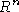is compactified to a unit hemisphere by stereographic projection, as discussed in Section , then the Euclidian metric takes the form (1) near the boundary.

A scattering metric is a, rather special, example of a metric on the structure bundle of the Lie algebra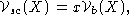where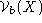is the Lie algebra of all smooth vector fields on X which are tangent to the boundary. The space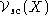is a boundary-fibration structure (which notion we do not define or use here) in the sense of daomwc, with structure bundle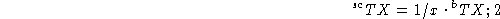this is discussed in Section S.Sa. The basic approach taken here and examined in some generality in daomwc is that the boundary-fibration structure, in this case just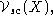should be thought of as a geometry. This approach is based on the development of a calculus of pseudodifferential operators, appropriate to the context and coming from the geometry. This calculus can then be applied, much as the usual calculus of pseudodifferential operators on a compact manifold without boundary can be applied, to give regularity, and in particular propagation, results.

Here the `small calculus' of scattering pseudodifferential operators is discussed and used to prove commutator estimates analogous to those found in scattering theory on Euclidian space. In particular we give a variant of Mourre's estimate (Mourre) in this setting. For the half-sphere,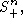this calculus reduces to one of the standard versions of the global pseudodifferential calculus on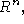via stereographic projection.

In fact three examples of boundary-fibration structures occur here; they can be recognized by the elliptic operators associated to each. Let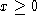be a coordinate defining the boundary (which is `at infinity') and let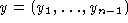be tangential coordinates. Then, with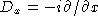and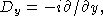typical (invertible) elliptic operators are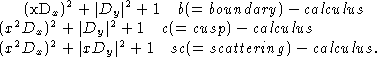Although the cusp calculus is not discussed in detail here, it would be natural to do so since its use makes the regularity results of Section S.Src more precise. Whereas the scattering calculus is tempered, in the sense that it is locally contained in the Weyl calculus of Hörmander HoWC, this is not the case for either the boundary or cusp calculi.

The material below falls naturally into three parts, the contents of which are described in more detail below. In Section sS.SpS.WF the quantization of the Lie algebra of vector fields associated to the metric (1) is explained. The central sections, Section sS.PtpS.Lrp contain the main analytic results, concerning the behaviour of the limit of the resolvent of the Laplacian on the spectrum and leading in particular to the definition of the scattering operator. In the third part, consisting of the appendices, the direct and intrinsic approach to the quantization is outlined.

The properties of the scattering operator in this generality remain to be investigated. In the Euclidian context, the scattering operator (at fixed energy) as defined in Section S.So is of the form R+A, where R is the anitpodal reflection of the sphere and A is a smoothing operator. In the general case of a scattering metric the appropriate generalization is expected to be:

Comjecture The scattering operator associated to a scattering metric is a Fourier integral operator with canonical relation the geodesic flow, at time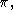of the boundary metric.

This can be construed as the statement that, for a metric (1), infinity always has widthOther topics which remain to be treated include:

1. a) The behaviour of the resolvent near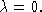This is considerably more complicated than in the Euclidian setting since it is influenced strongly by the eigenvalues of the induced Laplacian on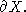2. b) The asymptotic behaviour of the resolvent kernel.
3. c) The derivation of a trace formula.

The central result proved below is that the-outgoing solution to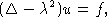wherevanishes with all its derivatives at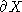andhas a complete asymptotic expansion with leading term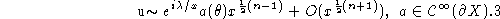This result, well known in the Euclidian setting, can be viewed as either a strong form of the Sommerfeld radiation condition or a weak form of b) above. The space of square-integrable functions with respect to the metric volume form is written here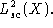Then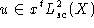for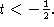Moreover iffor any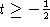then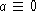and u vanishes rapidly atThe critical exponent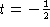is seen to arise from the commutator formula in the calculus of scattering pseudodifferential operators.

The structure of scattering differential operators is discussed in Section S.Sa, after it is shown in Section S.Sp that stereographic projection, i. e. radial compactification, ofconverts the translation invariance to such a structure. The properties of the Laplacian, acting on forms, associated to a metric (1), are examined in Section S.SL. The calculus of pseudodifferential operators obtained by quantization of the structure algebra is introduced in Section S.SD by reduction toand many of its properties are described in Section S.PC; although full proof are not given. Further discussion is relegated to Appendix A.Sc. In Section S.RF the properties of the calculus are used to show that the resolvent family for the Laplacian of (1) is holomorphic in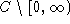with values in the calculus. The wavefront set associated to the scattering calculus, and a uniform version of it, is investigated in Section S.WF; this language is used as a convenient way to state microlocal estimates.

The first such estimates, reducing to Hörmander's propagation theorem for operators of real-principal type, are given in Section S.Ptp. The more subtle estimates, again proved by the positive-commutator method, around the radial surfaces for the operator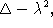are given in Section S.Rs. These are the main estimates used in the subsequent analysis and amount to a version in this context of Mourre's estimate for the N-body problem. In Section S.Aoee these basic estimates, together with a unique continuation theorem, or Aronszajn-Cordes type, proved by Hörmander (HoUC), show the absence of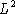eigenfunctions for the Laplacian. Various forms of the Sommerfeld radiation condition are discussed in Section S.Src and the asymptotic expansion of-outgoing solutions is deduced in Section S.Ae. The boundary pairing for asymptotic data in Section S.Bp is used in Section S.Lap to prove the limiting absorption principle, i. e. to examine the limit of the resolvent as the parameter approaches the spectrum. Finally in Section S.So these results are used to define the scattering operator for the Laplacian. In Section S.Lrp the minor modifications needed to treat long-range potentials are described.

The discussion below is intended to provide a bridge between the traditional methods of Euclidian scattering theory, which depends ultimately on the explicit inversion of the Euclidian Laplacian, and the more geometric problems encountered in the analysis of the Laplacian on complete Riemannian manifolds with uniform degeneracy at infinity, as typified by a metric singular at the boundary of a compact manifold with boundary. Indeed these scattering metrics form just such a class, but there are others of interest. Although all the results proved here are well known in the Euclidian setting we give proofs in this wider context. It is anticipated that the methods used here can be further generalized to give a unified treatment of the N-body problem and classes of singular metrics on manifolds with corners. For a recent discussion of the N-body problem see Yafaev.Next: About this document Up: No Title Previous: No Title

Richard B. Melrose
Sat Mar 30 08:28:47 EST 1996